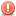CodeGuru Home VC++ / MFC / C++ .NET / C# Visual Basic VB Forums Developer.com

1.Junior MemberJoin Date
Aug 2011
Posts
1

##Master Methode

In master method they talk about functions being asymptotically larger and polynomialy larger.
But I don't feel much difference between them. What are their definitions ?

And in one case they say nlog2(n) is asymptotically larger than n but is not polynomialy larger than n . The reason they have given is that nlog(n)/n = log(n) is asymptotically less than n^e for any positive constant e. But for me the last part seems incorrect. Because for 0<e< 0.2 n^e is asymptotically smaller than log(n).
Am I correct?Reply With Quote

2.Junior MemberJoin Date
May 2011
Posts
22

## Re: Master Methode

Hi,

the whole asymtoticaly and polynominal-thing is much more show than really substance. There are a few Functions between log and polonoms and exp, but almost none of them has some real impact. (ok... maybe the iterated logarithm is sometimes important)
(thats my opinion)

For your question x^0.1 grows much faster than log(x). Just type very big numbers in your calculator. x^0.01 also grows faster than log(x). This is not a proof, just try around to get a feeling.

The proof for this goes like this:
If you paint x^n and exp(x) you will see, that exp(x) grows much faster than every x^n for each possible n in the natural Numbers. This is very easy to show, if you write exp(x)=1+x+x^2/2+x^2/6+ .... . Then you mirror the diagramms at the line x=y, then exp grows faster than every x^n is transformed to log(x) grows slower than every x^(1/n). 1/n can be smaller than every positive e. --> voila :-)

If you really want a proof with every details, you will get a few pages written with complicated formulas, but I hope the above gave some impression about the main points.

GMarcoReply With Quote

algorithm complexity, algorithms####Posting Permissions

• You may not post new threads
• You may not post replies
• You may not post attachments
• You may not edit your posts
•